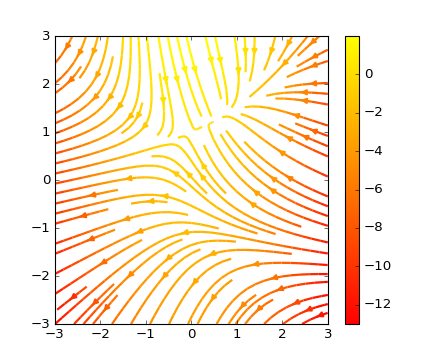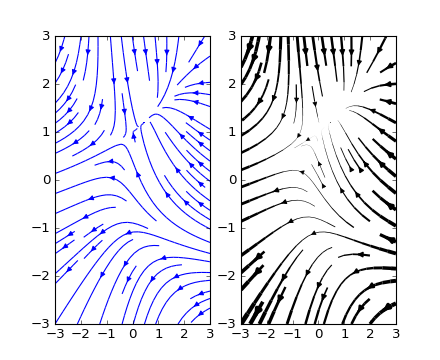### We're updating the default styles for Matplotlib 2.0

Learn what to expect in the new updates#### Previous topic

images_contours_and_fields example code: pcolormesh_levels.py

# images_contours_and_fields example code: streamplot_demo_features.py¶(png, hires.png, pdf)(png, hires.png, pdf)

```"""
Demo of the `streamplot` function.

A streamplot, or streamline plot, is used to display 2D vector fields. This
example shows a few features of the stream plot function:

* Varying the color along a streamline.
* Varying the density of streamlines.
* Varying the line width along a stream line.
"""
import numpy as np
import matplotlib.pyplot as plt

Y, X = np.mgrid[-3:3:100j, -3:3:100j]
U = -1 - X**2 + Y
V = 1 + X - Y**2
speed = np.sqrt(U*U + V*V)

fig0, ax0 = plt.subplots()
strm = ax0.streamplot(X, Y, U, V, color=U, linewidth=2, cmap=plt.cm.autumn)
fig0.colorbar(strm.lines)

fig1, (ax1, ax2) = plt.subplots(ncols=2)
ax1.streamplot(X, Y, U, V, density=[0.5, 1])

lw = 5*speed / speed.max()
ax2.streamplot(X, Y, U, V, density=0.6, color='k', linewidth=lw)

plt.show()
```

Keywords: python, matplotlib, pylab, example, codex (see Search examples)